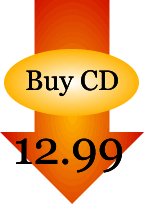# “12” Times Table

“You Can Multiply By Twelve” lyrics

If you can multiply by two (we do!)
And multiply by ten (we can!)
Then you can multiply by twelve right now
I'll show you how to begin

Just take the answer from two
When you multiply by twelve

If you can multiply by two (we do!)
And multiply by ten (we can!)
Then you can multiply by twelve right now
I'll show you how to begin

Just take the answer from two
When you multiply by twelve

So if you want to multiply 8 times 12,
Here's how to do it:

First, multiply 8 times 2
(That will give you 16)

And then multiply 8 times 10
(8 x 10 = 80)

And you'll get 96

(So 8 x 12 = 96?)

Every time! (I approve)

If you multiply by 2 and 10
You'll have multiplied by 12
You should be proud of yourself

When you multiply by 12

`© 2005 Power Arts Company, Inc.`CD Baby
iTunesSongs for Teaching
CD Baby
iTunesCD Baby

Reviews